# ECE 697 B 667 Spring 2006 Synthesis and

• Slides: 23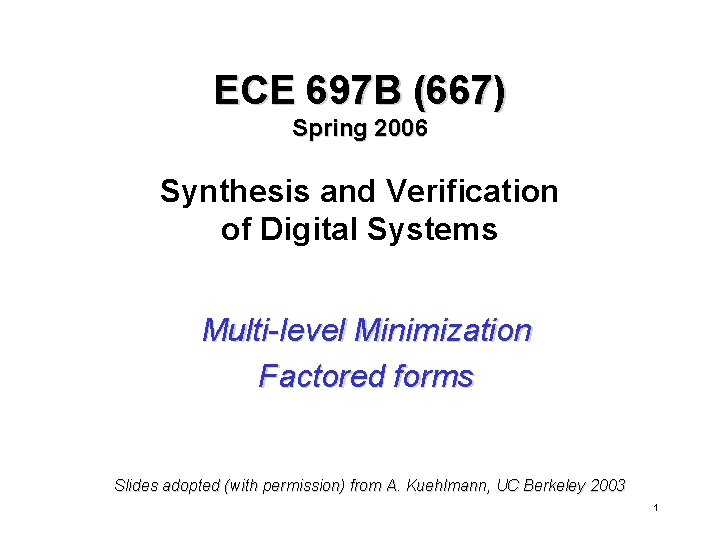ECE 697 B (667) Spring 2006 Synthesis and Verification of Digital Systems Multi-level Minimization Factored forms Slides adopted (with permission) from A. Kuehlmann, UC Berkeley 2003 1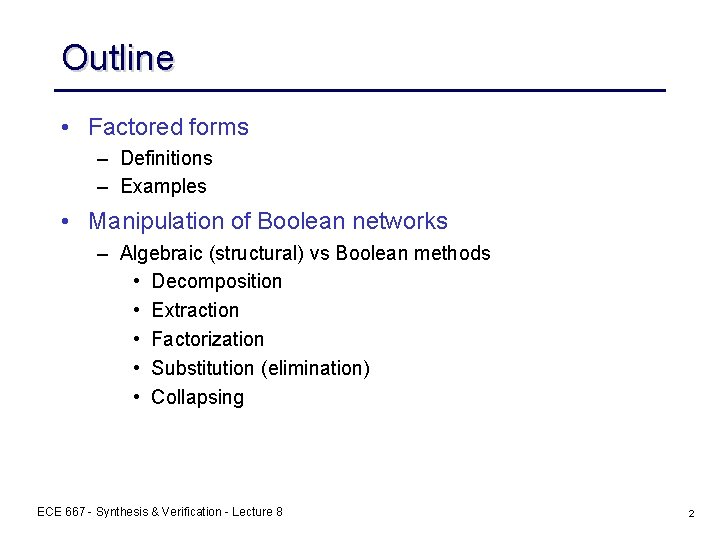Outline • Factored forms – Definitions – Examples • Manipulation of Boolean networks – Algebraic (structural) vs Boolean methods • Decomposition • Extraction • Factorization • Substitution (elimination) • Collapsing ECE 667 - Synthesis & Verification - Lecture 8 2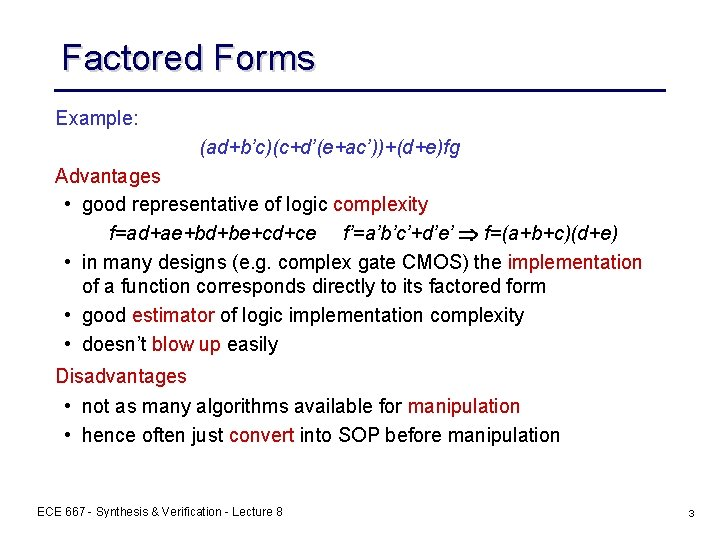Factored Forms Example: (ad+b’c)(c+d’(e+ac’))+(d+e)fg Advantages • good representative of logic complexity f=ad+ae+bd+be+cd+ce f’=a’b’c’+d’e’ f=(a+b+c)(d+e) • in many designs (e. g. complex gate CMOS) the implementation of a function corresponds directly to its factored form • good estimator of logic implementation complexity • doesn’t blow up easily Disadvantages • not as many algorithms available for manipulation • hence often just convert into SOP before manipulation ECE 667 - Synthesis & Verification - Lecture 8 3Factored Forms and CMOS Design Note: literal count » transistor count » area • however, area also depends on – wiring – gate size etc. • therefore a very crude measure ECE 667 - Synthesis & Verification - Lecture 8 4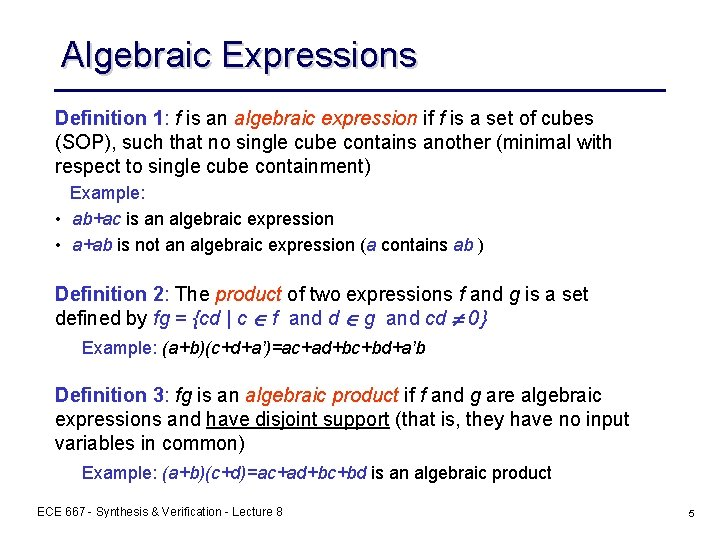Algebraic Expressions Definition 1: f is an algebraic expression if f is a set of cubes (SOP), such that no single cube contains another (minimal with respect to single cube containment) Example: • ab+ac is an algebraic expression • a+ab is not an algebraic expression (a contains ab ) Definition 2: The product of two expressions f and g is a set defined by fg = {cd | c f and d g and cd 0} Example: (a+b)(c+d+a’)=ac+ad+bc+bd+a’b Definition 3: fg is an algebraic product if f and g are algebraic expressions and have disjoint support (that is, they have no input variables in common) Example: (a+b)(c+d)=ac+ad+bc+bd is an algebraic product ECE 667 - Synthesis & Verification - Lecture 8 5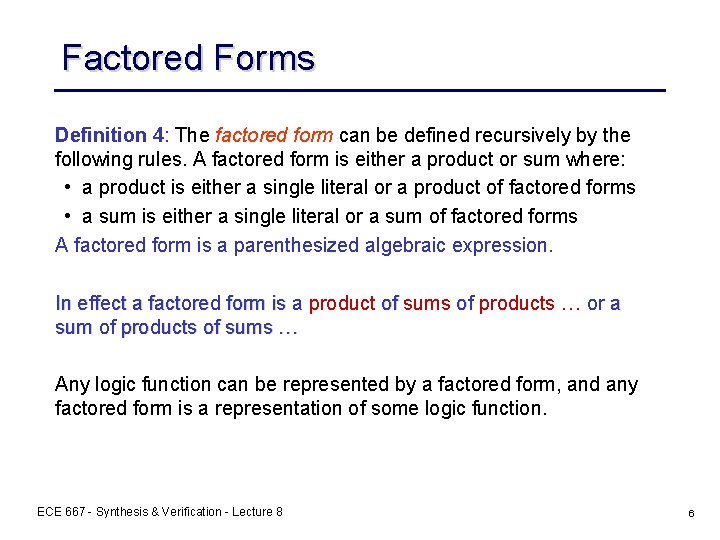Factored Forms Definition 4: The factored form can be defined recursively by the following rules. A factored form is either a product or sum where: • a product is either a single literal or a product of factored forms • a sum is either a single literal or a sum of factored forms A factored form is a parenthesized algebraic expression. In effect a factored form is a product of sums of products … or a sum of products of sums … Any logic function can be represented by a factored form, and any factored form is a representation of some logic function. ECE 667 - Synthesis & Verification - Lecture 8 6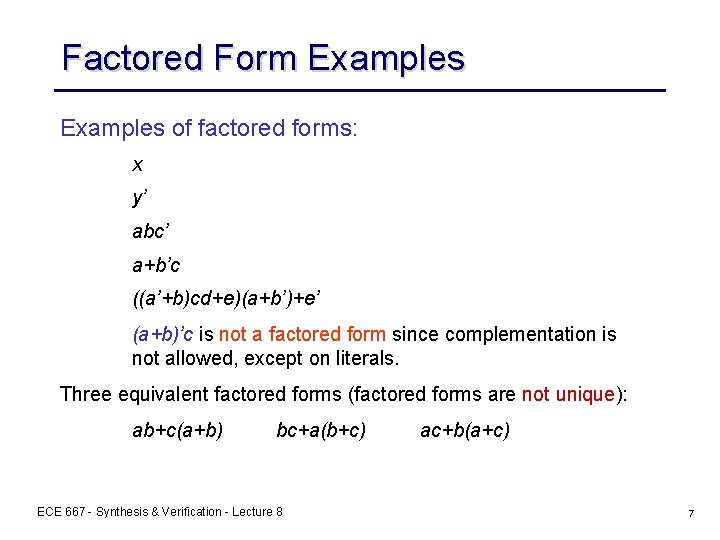Factored Form Examples of factored forms: x y’ abc’ a+b’c ((a’+b)cd+e)(a+b’)+e’ (a+b)’c is not a factored form since complementation is not allowed, except on literals. Three equivalent factored forms (factored forms are not unique): ab+c(a+b) bc+a(b+c) ECE 667 - Synthesis & Verification - Lecture 8 ac+b(a+c) 7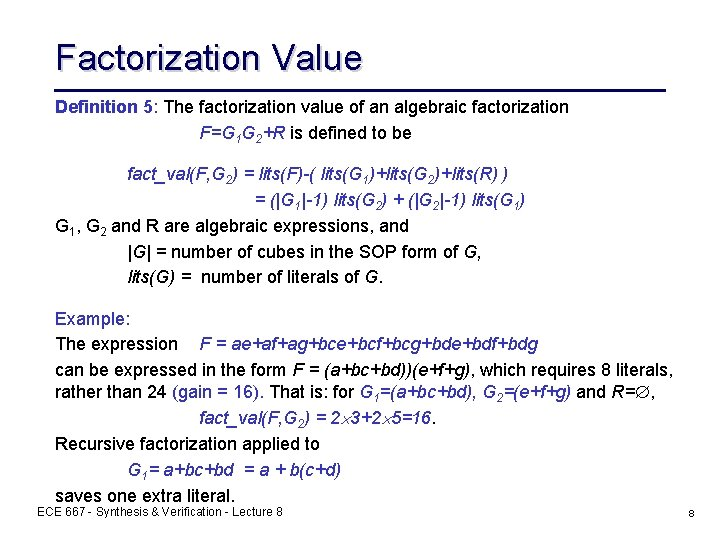Factorization Value Definition 5: The factorization value of an algebraic factorization F=G 1 G 2+R is defined to be fact_val(F, G 2) = lits(F)-( lits(G 1)+lits(G 2)+lits(R) ) = (|G 1|-1) lits(G 2) + (|G 2|-1) lits(G 1) G 1, G 2 and R are algebraic expressions, and |G| = number of cubes in the SOP form of G, lits(G) = number of literals of G. Example: The expression F = ae+af+ag+bce+bcf+bcg+bde+bdf+bdg can be expressed in the form F = (a+bc+bd))(e+f+g), which requires 8 literals, rather than 24 (gain = 16). That is: for G 1=(a+bc+bd), G 2=(e+f+g) and R= , fact_val(F, G 2) = 2 3+2 5=16. Recursive factorization applied to G 1= a+bc+bd = a + b(c+d) saves one extra literal. ECE 667 - Synthesis & Verification - Lecture 8 8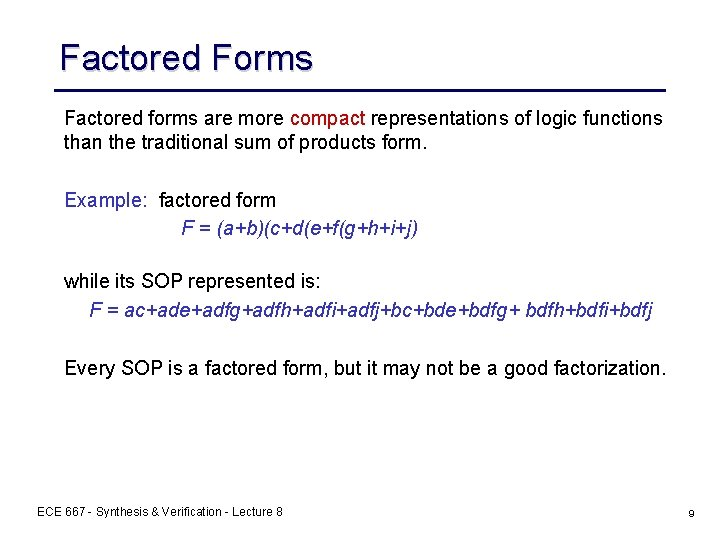Factored Forms Factored forms are more compact representations of logic functions than the traditional sum of products form. Example: factored form F = (a+b)(c+d(e+f(g+h+i+j) while its SOP represented is: F = ac+ade+adfg+adfh+adfi+adfj+bc+bde+bdfg+ bdfh+bdfi+bdfj Every SOP is a factored form, but it may not be a good factorization. ECE 667 - Synthesis & Verification - Lecture 8 9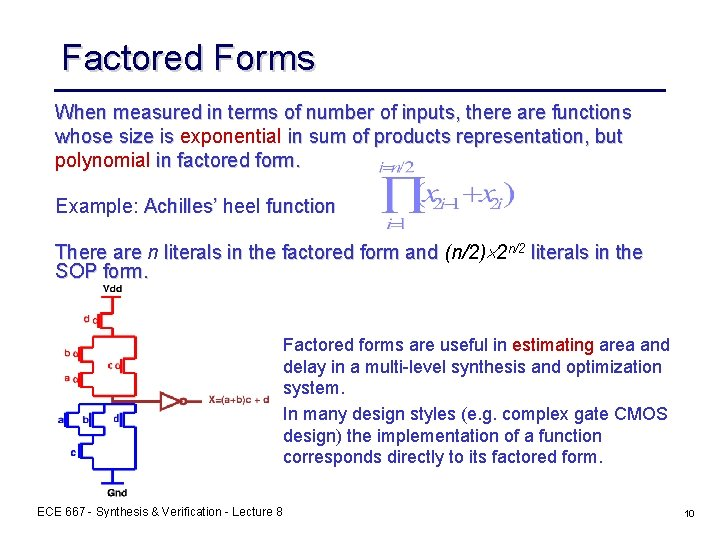Factored Forms When measured in terms of number of inputs, there are functions whose size is exponential in sum of products representation, but polynomial in factored form. Example: Achilles’ heel function There are n literals in the factored form and (n/2) 2 n/2 literals in the SOP form. Factored forms are useful in estimating area and delay in a multi-level synthesis and optimization system. In many design styles (e. g. complex gate CMOS design) the implementation of a function corresponds directly to its factored form. ECE 667 - Synthesis & Verification - Lecture 8 10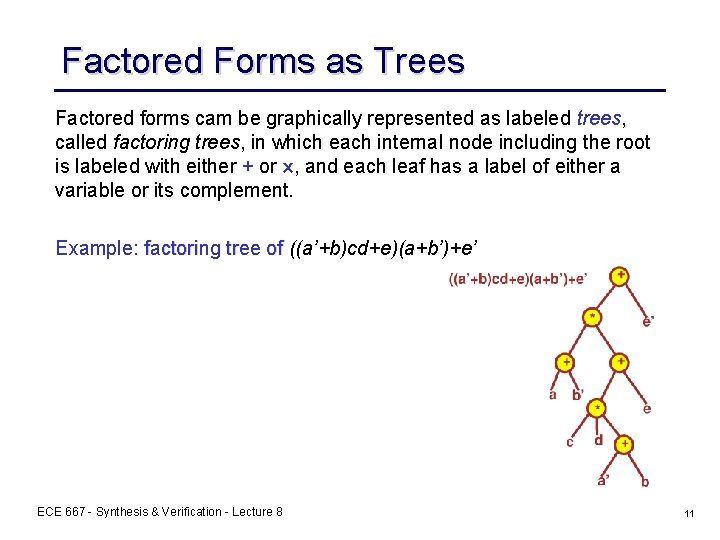Factored Forms as Trees Factored forms cam be graphically represented as labeled trees, called factoring trees, in which each internal node including the root is labeled with either + or , and each leaf has a label of either a variable or its complement. Example: factoring tree of ((a’+b)cd+e)(a+b’)+e’ ECE 667 - Synthesis & Verification - Lecture 8 11Factored Forms - Size Definition 6: The size of a factored form F (denoted (F )) is the number of literals in the factored form. Example: (( a+b)ca’) = 4 ((a+b+cd)(a’+b’)) =6 (( • A factored form is optimal if no other factored form (for that function) has fewer literals. • A factored form is positive unate in x, if x appears in F, but x’ does not. • A factored form is negative unate in x, if x’ appears in F, but x does not. • F is unate in x if it is either positive or negative unate in x, otherwise F is binate in x. Example: (a+b’)c+a’ is positive unate in c, negative unate in b, and binate in a. ECE 667 - Synthesis & Verification - Lecture 8 12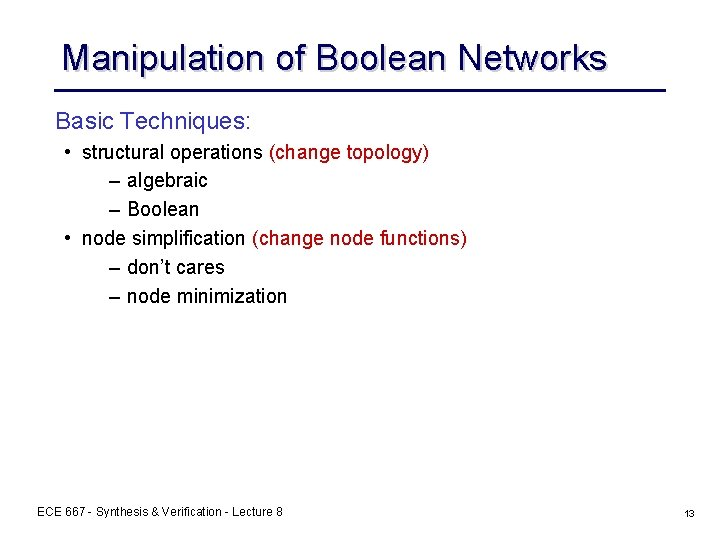Manipulation of Boolean Networks Basic Techniques: • structural operations (change topology) – algebraic – Boolean • node simplification (change node functions) – don’t cares – node minimization ECE 667 - Synthesis & Verification - Lecture 8 13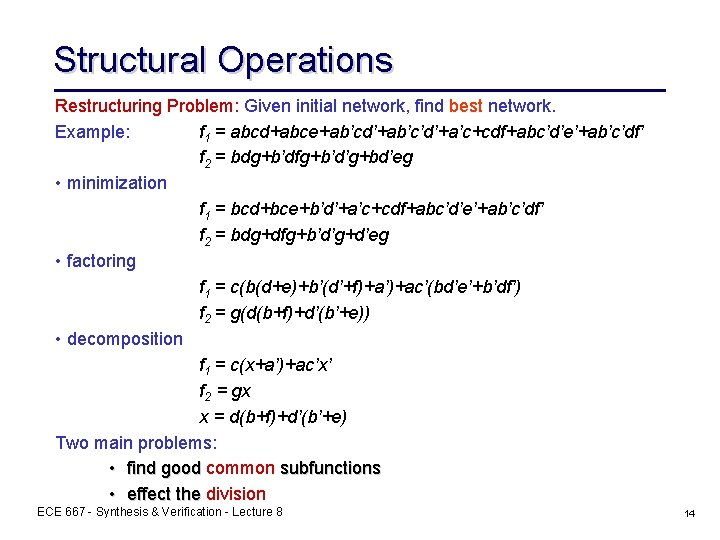Structural Operations Restructuring Problem: Given initial network, find best network. Example: f 1 = abcd+abce+ab’cd’+ab’c’d’+a’c+cdf+abc’d’e’+ab’c’df’ f 2 = bdg+b’dfg+b’d’g+bd’eg • minimization f 1 = bcd+bce+b’d’+a’c+cdf+abc’d’e’+ab’c’df’ f 2 = bdg+dfg+b’d’g+d’eg • factoring f 1 = c(b(d+e)+b’(d’+f)+a’)+ac’(bd’e’+b’df’) f 2 = g(d(b+f)+d’(b’+e)) • decomposition f 1 = c(x+a’)+ac’x’ f 2 = gx x = d(b+f)+d’(b’+e) Two main problems: • find good common subfunctions • effect the division ECE 667 - Synthesis & Verification - Lecture 8 14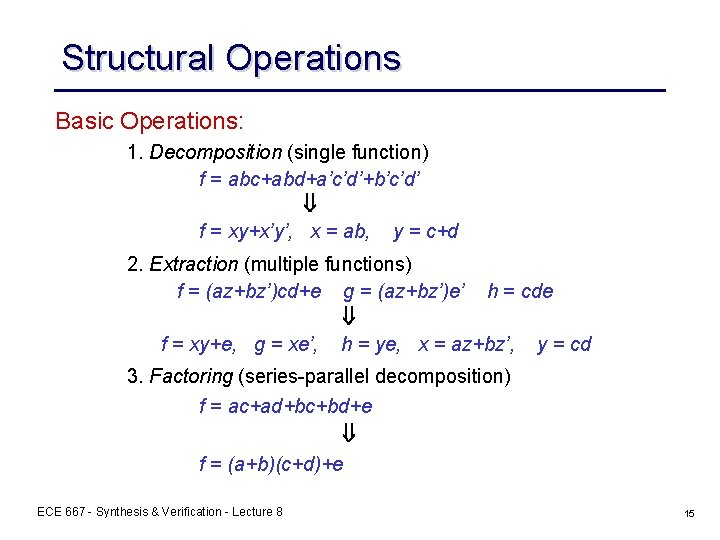Structural Operations Basic Operations: 1. Decomposition (single function) f = abc+abd+a’c’d’+b’c’d’ f = xy+x’y’, x = ab, y = c+d 2. Extraction (multiple functions) f = (az+bz’)cd+e g = (az+bz’)e’ h = cde f = xy+e, g = xe’, h = ye, x = az+bz’, y = cd 3. Factoring (series-parallel decomposition) f = ac+ad+bc+bd+e f = (a+b)(c+d)+e ECE 667 - Synthesis & Verification - Lecture 8 15Structural Operations 4. Substitution g = a+b f = ac+bc + d f = gc+d 5. Collapsing (also called elimination) f = ga+g’b g = c+d f = ac+ad+bc’d’ Note: division plays a key role in all of these ECE 667 - Synthesis & Verification - Lecture 8 16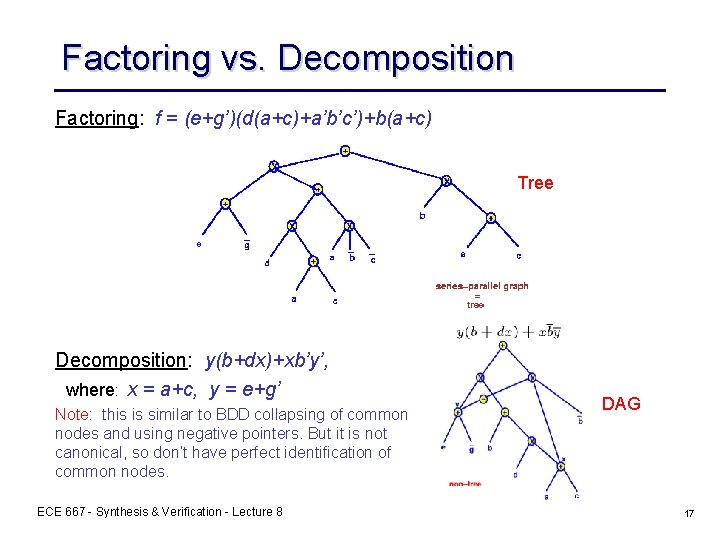Factoring vs. Decomposition Factoring: f = (e+g’)(d(a+c)+a’b’c’)+b(a+c) Tree Decomposition: y(b+dx)+xb’y’, where: x = a+c, y = e+g’ Note: : this is similar to BDD collapsing of common nodes and using negative pointers. But it is not canonical, so don’t have perfect identification of common nodes. ECE 667 - Synthesis & Verification - Lecture 8 DAG 17Value of Node Elimination These nodes are expressed in terms of node yj (contain literal yj) ni = number of times literal yj or yj’ occurs in factored form fi lj = number of literals in factored form fj No. of literals with factoring: No. of literals without factoring: • value (gain) of node j = cost(without factoring) - cost(with factoring) Note: we can treat yj and yj’ the same way since ( Fj ) = ( Fj’ ). ECE 667 - Synthesis & Verification - Lecture 8 18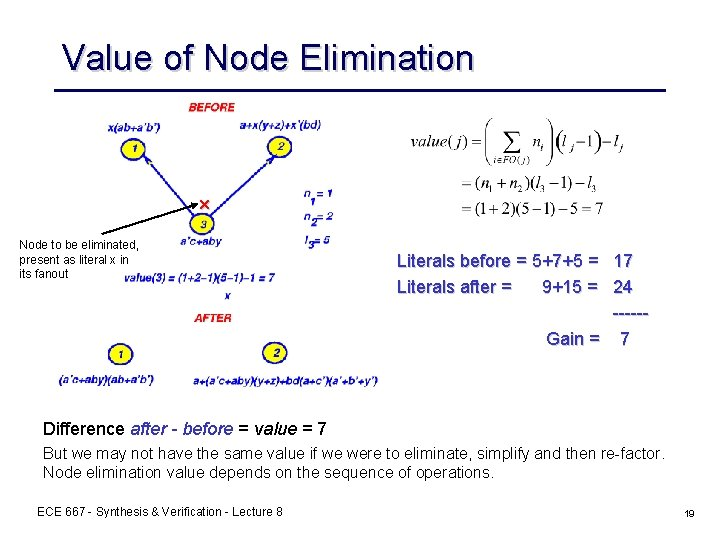Value of Node Elimination x Node to be eliminated, present as literal x in its fanout Literals before = 5+7+5 = 17 Literals after = 9+15 = 24 -----Gain = 7 Difference after - before = value = 7 But we may not have the same value if we were to eliminate, simplify and then re-factor. Node elimination value depends on the sequence of operations. ECE 667 - Synthesis & Verification - Lecture 8 19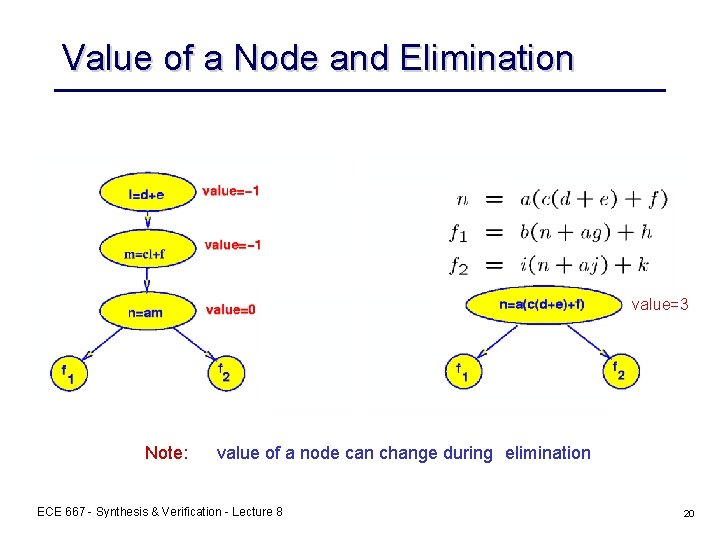Value of a Node and Elimination value=3 Note: value of a node can change during elimination ECE 667 - Synthesis & Verification - Lecture 8 20Optimum Factored Forms • Definition 7: – Let f be a completely specified Boolean function, and (f) be the minimum number of literals in any factored form of f. • Definition 8: – Let sup(f) be the true variable support of f, i. e. the set of variables f depends on. Two functions f and g are orthogonal (f g), if sup(f) sup(g)=. ECE 667 - Synthesis & Verification - Lecture 8 21Optimum Factored Forms Lemma: Let f=g+h such that g h, then (f)= (g)+ (h). Proof: Let F, G and H be the optimum factored forms of f, g and h. Since G+H is a factored form, (f)= (F) (G+H)= (g)+ (h). Let c be a minterm, on sup(g), of g’. Since g and h have disjoint support, we have fc=(g+h)c=gc+hc=0+hc=hc=h. Similarly, if d is a minterm of h’, fd=g. Because (h)= (fc) (Fc) and (g)= (fd) (Fd), (h)+ (g) (Fc)+ (Fd). Let m (n) be the number of literals in F that are from SUPPORT(g) (SUPPORT(h)). When computing Fc (Fd), we replace all the literals from SUPPORT(g) (SUPPORT(h)) by the appropriate values and simplify the factored form by eliminating all the constants and possibly some literals from sup(g) (sup(h)) by using the Boolean identities. Hence (Fc) n and (Fd) m. Since (F)=m+n, (Fc)+ (Fd) m+n= (F). We have (f) (g)+ (h) (Fc)+ (Fd) (F) (f)= (F). ECE 667 - Synthesis & Verification - Lecture 8 22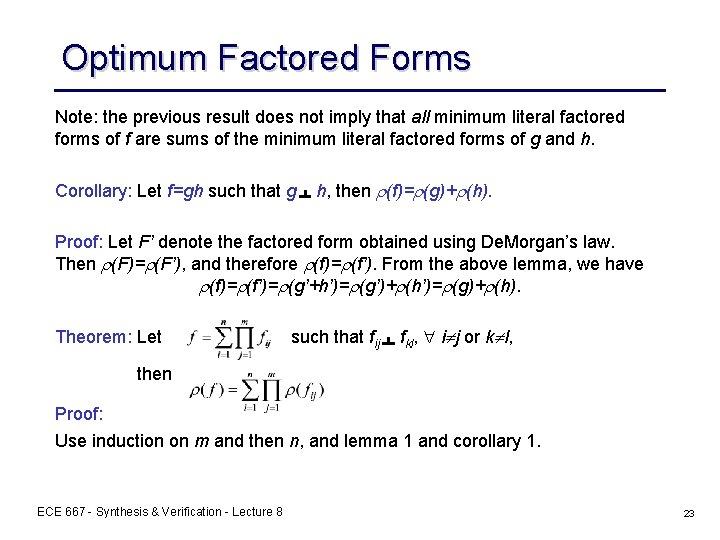Optimum Factored Forms Note: the previous result does not imply that all minimum literal factored forms of f are sums of the minimum literal factored forms of g and h. Corollary: Let f=gh such that g h, then (f)= (g)+ (h). Proof: Let F’ denote the factored form obtained using De. Morgan’s law. Then (F)= (F’), and therefore (f)= (f’). From the above lemma, we have (f)= (f’)= (g’+h’)= (g’)+ (h’)= (g)+ (h). Theorem: Let such that fij fkl, i j or k l, then Proof: Use induction on m and then n, and lemma 1 and corollary 1. ECE 667 - Synthesis & Verification - Lecture 8 23